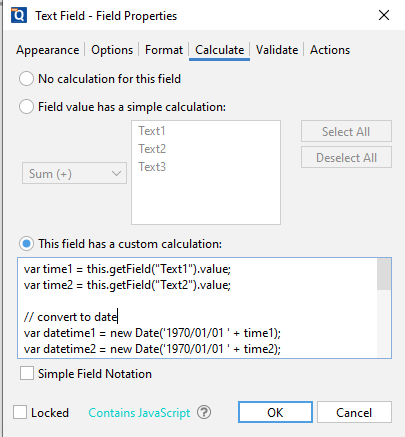Q: I want to create a new form field using custom JavaScript calculation that is the difference in time between 2 other interactive form fields containing time value. Can you provide an example for that?

A: Here is a simple example to add custom JavaScript calculations to calculate date in a PDF. In this article, we have 2 text fields “Text1”, “Text2” and we want to show the difference in time in a 3rd text field “Text3” in hours and minutes.

1. In PDF Studio, go to the “Forms” tab and click on Text Field

2. Create three text fields called Text1, Text2 and Text3.

3. Right click on field Text3 and select Properties

4. Click Calculate and select “This field has a custom calculations”

5. Put the following script under text box:

var time1 = this.getField(“Text1”).value;
var time2 = this.getField(“Text2”).value;

// convert to date
var datetime1 = new Date(‘1970/01/01 ‘ + time1);
var datetime2 = new Date(‘1970/01/01 ‘ + time2);

var diffInMilliSeconds = Math.abs(datetime1 – datetime2) / 1000;

// calculate hours
var hours = Math.floor(diffInMilliSeconds / 3600) % 24;
diffInMilliSeconds -= hours * 3600;

// calculate minutes
var minutes = Math.floor(diffInMilliSeconds / 60) % 60;
diffInMilliSeconds -= minutes * 60;

// set field value to the difference
event.value =hours + “:” +minutes;7. Hit OK

8. Exit form editing (by clicking on the Home Tab)

9. Start testing the calculations.